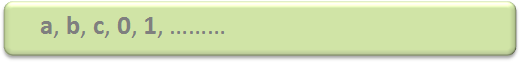Open in App
Not now

# Introduction of Theory of Computation

• Difficulty Level : Easy
• Last Updated : 19 Jul, 2022

Automata theory (also known as Theory Of Computation) is a theoretical branch of Computer Science and Mathematics, which mainly deals with the logic of computation with respect to simple machines, referred to as automata.

Automata* enables scientists to understand how machines compute the functions and solve problems. The main motivation behind developing Automata Theory was to develop methods to describe and analyze the dynamic behavior of discrete systems.

Automata originated from the word “Automaton” which is closely related to “Automation”.

### Basic Terminologies of Theory of Computation:

Now, let’s understand the basic terminologies, which are important and frequently used in the Theory of Computation.

### Symbol:

A symbol (often also called a character) is the smallest building block, which can be any alphabet, letter, or picture.### Alphabets (Σ):

Alphabets are a set of symbols, which are always finite### String:

A string is a finite sequence of symbols from some alphabet. A string is generally denoted as w and the length of a string is denoted as |w|

```Empty string is the string with
zero occurrence of symbols,
represented as ε.```
```Number of Strings (of length 2)
that can be generated over the alphabet {a, b}:
-   -
a   a
a   b
b   a
b   b

Length of String |w| = 2
Number of Strings = 4

Conclusion:
For alphabet {a, b} with length n, number of
strings can be generated = 2n.```

Note: If the number of symbols in the alphabet Σ is represented by |Σ|, then a number of strings of length n, possible over Σ is |Σ|n.

### Closure Representation in TOC:

L+: It is a Positive Closure that represents a set of all strings except Null or ε-strings.

L*: It is “Kleene Closure“, that represents the occurrence of certain alphabets for given language alphabets from zero to the infinite number of times. In which ε-string is also included.

From the above two statements, it can be concluded that:

`L* = εL+`
```Example:
(a) Regular expression for language accepting all combination of g's over Σ={g}:
R = g*
R={ε,g,gg,ggg,gggg,ggggg,...}

(b) Regular Expression for language accepting all combination of g's over Σ={g} :
R = g+
R={g,gg,ggg,gggg,ggggg,gggggg,...}```

Note: Σ* is a set of all possible strings(often power set(need not be unique here or we can say multiset) of string) So this implies that language is a subset of Σ*.This is also called a “Kleene Star”.

Kleene Star is also called a “Kleene Operator” or “Kleene Closure”. Engineers and IT professionals make use of Kleene Star to achieve all set of strings which is to be included from a given set of characters or symbols. It is one kind of Unary operator. In Kleene Star methodology all individual elements of a given string must be present but additional elements or combinations of these alphabets can be included to any extent.

```Example:
Input String: "GFG".
Σ* = { ε,"GFG","GGFG","GGFG","GFGGGGGGGG","GGGGGGGGFFFFFFFFFGGGGGGGG",...}
(Kleene Star is an infinite set but if we provide any grammar rules then it can work as a finite set.
Please note that we can include ε string also in given Kleene star representation.)```

### Language:

A language is a set of strings, chosen from some Σ* or we can say- A language is a subset of Σ* . A language that can be formed over ‘ Σ ‘ can be Finite or Infinite.

```Example of Finite Language:
L1 = { set of string of 2 }
L1 = { xy, yx, xx, yy }

Example of Infinite Language:
L1 = { set of all strings starts with 'b' }
L1 = { babb, baa, ba, bbb, baab, ....... }```
My Personal Notes arrow_drop_up
Related Articles